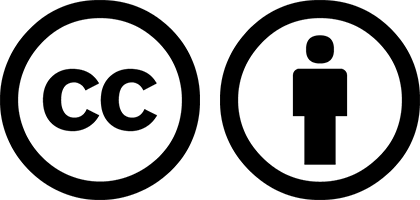# Powershell 修改提示符的文字内容和颜色PowerShell 自带的提示符不够美观，影响工作效率。本文介绍一种自定义 PowerShell 命令提示符的方法。

## 效果展示## ps1 文件

`ps1` 文件类似 linux 的 `~/.profile` 文件，在 shell 初始化时会预先执行。在 Powershell 中， `ps1` 文件的路径保存在 `\$Profile` 变量中，输入 `echo \$Profile` 命令能看到它的绝对路径：## prompt 函数

Powershell 有一个特殊的函数： `prompt` 函数，这个函数规定了提示符的内容和外观。默认的 `prompt` 函数规定了提示符的默认外观，也就是这样：## 查看当前使用的 prompt 函数

``````(Get-Command Prompt).ScriptBlock
``````## prompt 函数的基本格式

prompt 函数 的基本格式为：

``````function Prompt {
# 编辑你的内容
return ""
}
``````

## 修改 prompt 函数

### 固定字符串

``````function Prompt {
"R |"
}
``````### 函数返回值

``````function Prompt {
"\$(Get-Date)> "
}
``````## 使修改生效

``````. \$Profile
``````

``````function Prompt {
"R |"
}
``````

``````function Prompt {
"V -"
}
``````## 具体实现

### 步骤思路

1. 获取当前路径
2. 获取用户主目录
3. 将当前路径中的主目录部分，替换为波浪线（~）
4. 将替换后的结果加上颜色

### 1. 获取当前路径

``````\$promptString = "\$(Get-Location)"
``````

### 2. 获取用户主目录

``````\$homepath = \$env:userprofile
``````

``````\$pattern = \$homepath -replace '\\', '\\'
``````

### 3. 将当前路径中的主目录部分，替换为波浪线（~）

``````\$promptString = \$promptString -replace \$pattern, "~"
``````

`C:\Users\name\some\path` 这样的路径被替换为 `~\some\path`

### 4. 将替换后的结果加上颜色

``````return "`e[1;32m" + \$promptString + "`e[m "
``````

### 5. 合成到一起作为函数体

``````function Prompt {
\$promptString = "\$(Get-Location)"
\$homepath = \$env:userprofile
\$pattern = \$homepath -replace '\\', '\\'
\$promptString = \$promptString -replace \$pattern, "~"
return "`e[1;32m" + \$promptString + "`e[m "
}
``````

``````function Prompt {
\$pattern = \$env:userprofile -replace '\\', '\\'
\$promptString = "\$(Get-Location)" -replace \$pattern, "~"
"`e[1;32m" + \$promptString + "`e[m "
}
``````

## 调试## 显示 git 信息